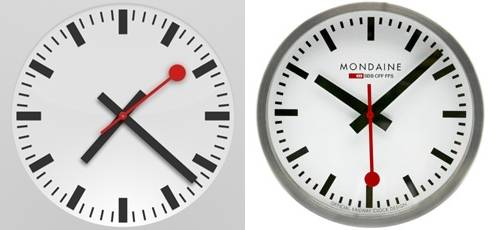# Swiss Railway Clock in HaskellI wanted a small Haskell programming task to solve. I had just read about the controversy around the new Clock application in iOS 6 which is trademarked by the Swiss Federal Railway (see Swiss Railway Clock (Wikipedia)). The clock design is simple and elegant and I though that this would be a nice task to re-implement in Haskell.

I have used gloss for some projects before so this was my first choice to output some graphics. The project was small enough (58 LOC) to be completed in a few evenings.

• I started by measuring the different clock elements from a screenshot of the iOS application.
• Then I defined new types for the clocks hands and time marks which comprised the different characteristics.
• It turns out that most elements are just simple radial lines (RLine) through the center of the clock. draw_line handles all drawing except the dots on the second hand.
• Gloss support simple animations where all you need to do is to provide is a simple function which produce a Picture at a certain time (in seconds) after the program was started. See Graphics.Gloss.Interface.IO.Animate.
• So I get the initial system clock and time zone and feed that into my clock graphics generation function.

This is the resulting code.

module Main (main) where
import Graphics.Gloss
import System.Time

type Len = Float
type Angle = Float
type SubSecond = Float
data Face = Face { r_face :: Len, thick :: Len }        -- Center is assumed at origo
data RLine = RLine { len :: Len, width :: Len, r_tip :: Len, col :: Color }
newtype Mark = MarkC { mRLine :: RLine }
newtype Hand = HandC { hRLine :: RLine }
data SecHand = SecHandC { hand :: RLine, r_cent :: Len, r_outer :: Len }

-- Draw RLine at a certain angle. These are drawn through the center of the faceto r_tip.
-- If len > r_tip then the line passes through origo. Angle 0 is represented at 12 a clock direction.
draw_line :: RLine -> Angle -> Picture
draw_line (RLine len width r_tip col) a = Color col $Rotate a$ Translate 0 (-len/2+r_tip) $rectangleSolid width len where -- Draw clock face with a surrounding circle at radius, r and hour and minute marks draw_face :: Face -> Mark -> Mark -> Picture draw_face (Face r w) (MarkC h) (MarkC m) = pictures$ face ++ hour_marks ++ min_marks where
hour_marks = map (\a -> draw_line h a) [0,30..330]
min_marks = map (\a -> draw_line m a) [0,6..354]    -- TODO: Rem. hour marks
face = [ThickCircle r w]

-- Draw clock hands according to calendar time, t
draw_hands :: ClockTime -> SubSecond -> Hand -> Hand -> SecHand -> Picture
draw_hands ct ss (HandC h) (HandC m) (SecHandC s r1 r2) = Color (col s) $pictures$ hour ++ min ++ sec ++ dots where
t = toUTCTime ct
seconds = ss + (fromIntegral $ctSec t) -- set ss=0 if you want distinct seconds minutes = fromIntegral$ ctMin t
hours = fromIntegral $ctHour t hour = [draw_line h (30 * (hours + minutes/60))] min = [draw_line m (6 * minutes)] sec = [draw_line s (6 * seconds)] dots = [circleSolid r1, Translate sec_x sec_y (circleSolid r2)] sec_x = (r_tip s) * cos(sec_radians) sec_y = (r_tip s) * sin(sec_radians) sec_radians = (pi/2) - (pi/30) * seconds -- Get current local time zone diff in hours get_timezone :: ClockTime -> IO Int get_timezone ct = do cal <- toCalendarTime ct return ((ctTZ cal) div 3600) -- Draw clock with clock time (ct) and timezone (tz) (hours) and a time diff (td) (seconds) since start drawClock :: ClockTime -> Int -> Float -> Picture drawClock ct tz td = Translate trans trans$ Scale scale scale $picture where size = 600 scale = 0.4 trans = -0.0*(fromIntegral size) picture = pictures [draw_face face m_hour m_min, draw_hands ct' subsec h_hour h_min h_sec] -- Ugly way to handle timezone. This works because draw_hands use toUTCTime ct' = addToClockTime (TimeDiff 0 0 0 tz 0 sec 0) ct (sec, subsec) = properFraction td face = Face 714 5 m_hour = MarkC$ RLine 158 54 690 black
m_min = MarkC $RLine 68 12 690 black h_hour = HandC$ RLine 590 54 386 black
h_min = HandC \$ RLine 826 54 622 black
h_sec = SecHandC (RLine 626 16 445 red) 23 55

main = do
ct <- getClockTime
tz <- get_timezone ct
animate
(InWindow "Swiss Railway Clock by Apple" (600,600) (100,100))
white                   -- background color
(drawClock ct tz)       -- picture to display

A screenshot of the resulting clock shown below. Unfortunately gloss does not yet support anti-aliasing which would make the graphics more smooth.I have generated a binary application for MacOS Lion.

• AppleClock?

To generate a new binary i used the following commands.

ghc -O2 -dylib-install-name /Applications/Xcode.app/Contents/Developer//Platforms/MacOSX.platform/Developer/SDKs/MacOSX10.7.sdk/usr/lib AppleClock.hs
strip AppleClock -o AppleClockStrip

./AppleClockStrip